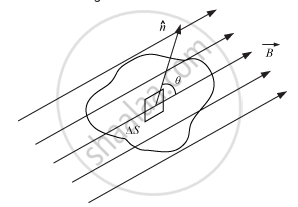# Answer the Following Question. State Gauss'S Law for Magnetism. Explain Its Significance. - Physics

State Gauss's law for magnetism. Explain its significance.

#### Solution

The net magnetic flux (ΦB) through any closed surface is always zero.

This law suggests that the number of magnetic field lines leaving any closed surface is always equal to the number of magnetic field lines entering it.Suppose a closed surface S is held in a uniform magnetic field vecB.

Consider a small vector area element Δvec"S" of this surface.

Magnetic flux through this area element is defined as ΔΦB = vec"B".Δvec"S"

Considering all small area elements of the surface, we obtain net magnetic flux through the surface as:

ØB = sum_"All" ΔØ_"B"  = sum_"All" vec"B". Δvec"S" = 0

Concept: Gauss’s Law
Is there an error in this question or solution?